Question

How to solve this question? By using the signal flow graph and the Mason Rule, derive...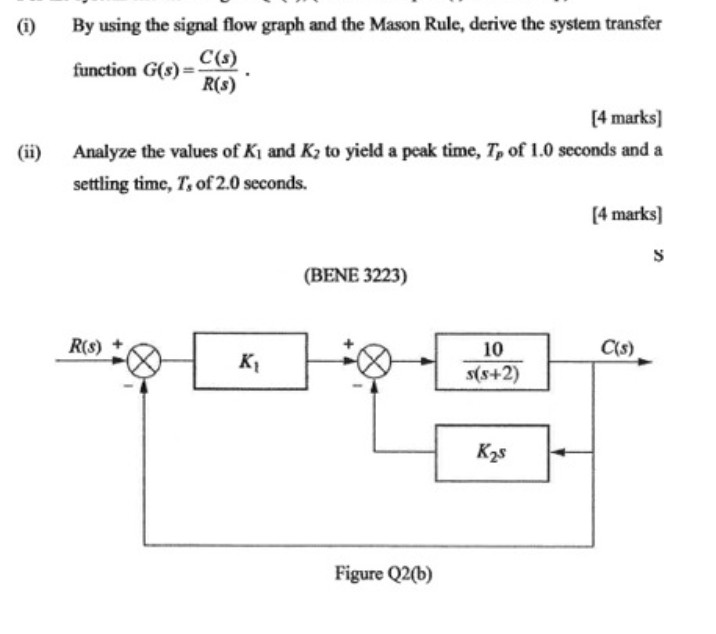How to solve this question?

By using the signal flow graph and the Mason Rule, derive the system transfer C(s) function G(s)= R(S) [4 marks) Analyze the values of Ki and Ky to yield a peak time, Tp of 1.0 seconds and a settling time, T. of 2.0 seconds. [4 marks) (ii) S (BENE 3223) R(s) C(s) K 10 s(s+2) Kas Figure Q2(b)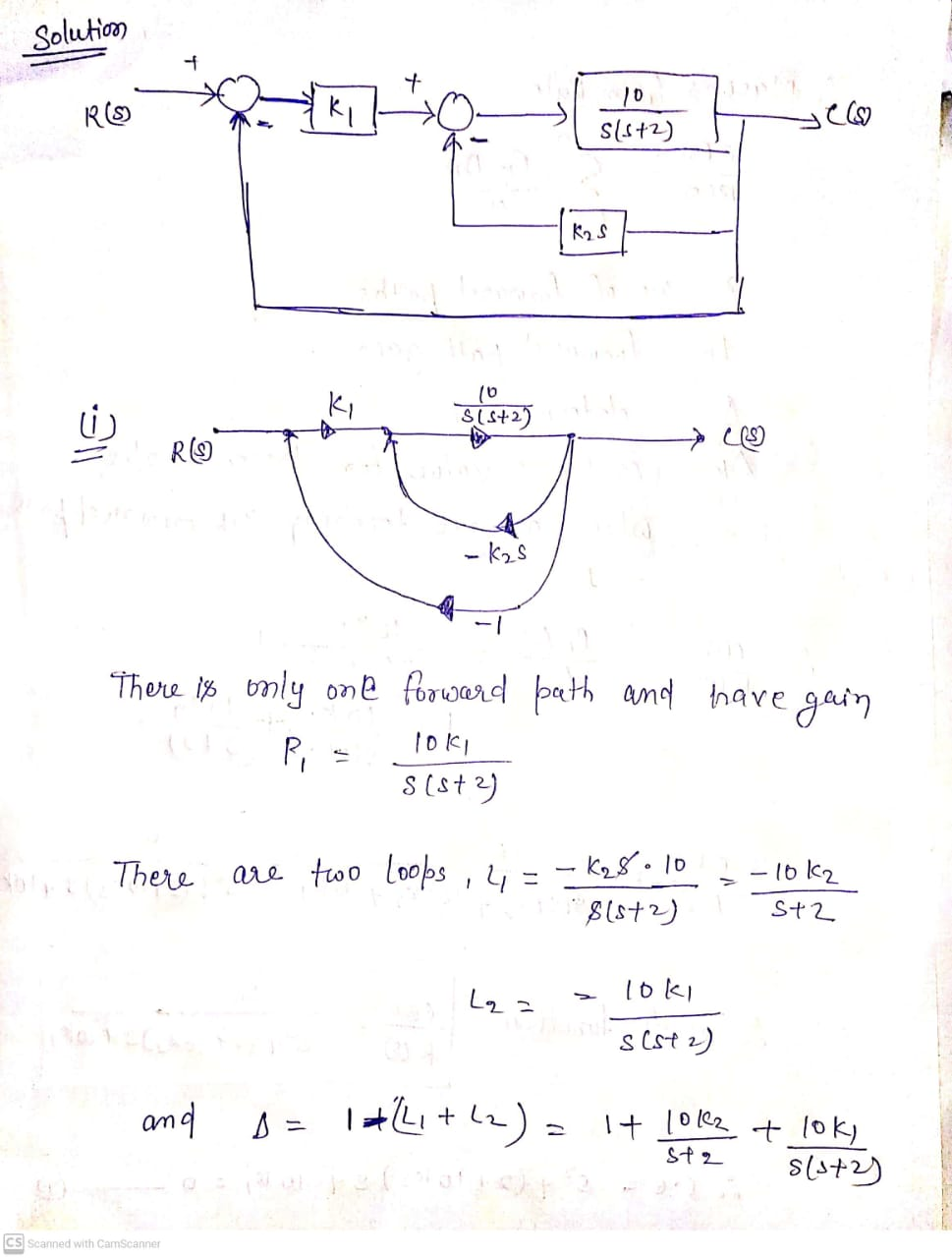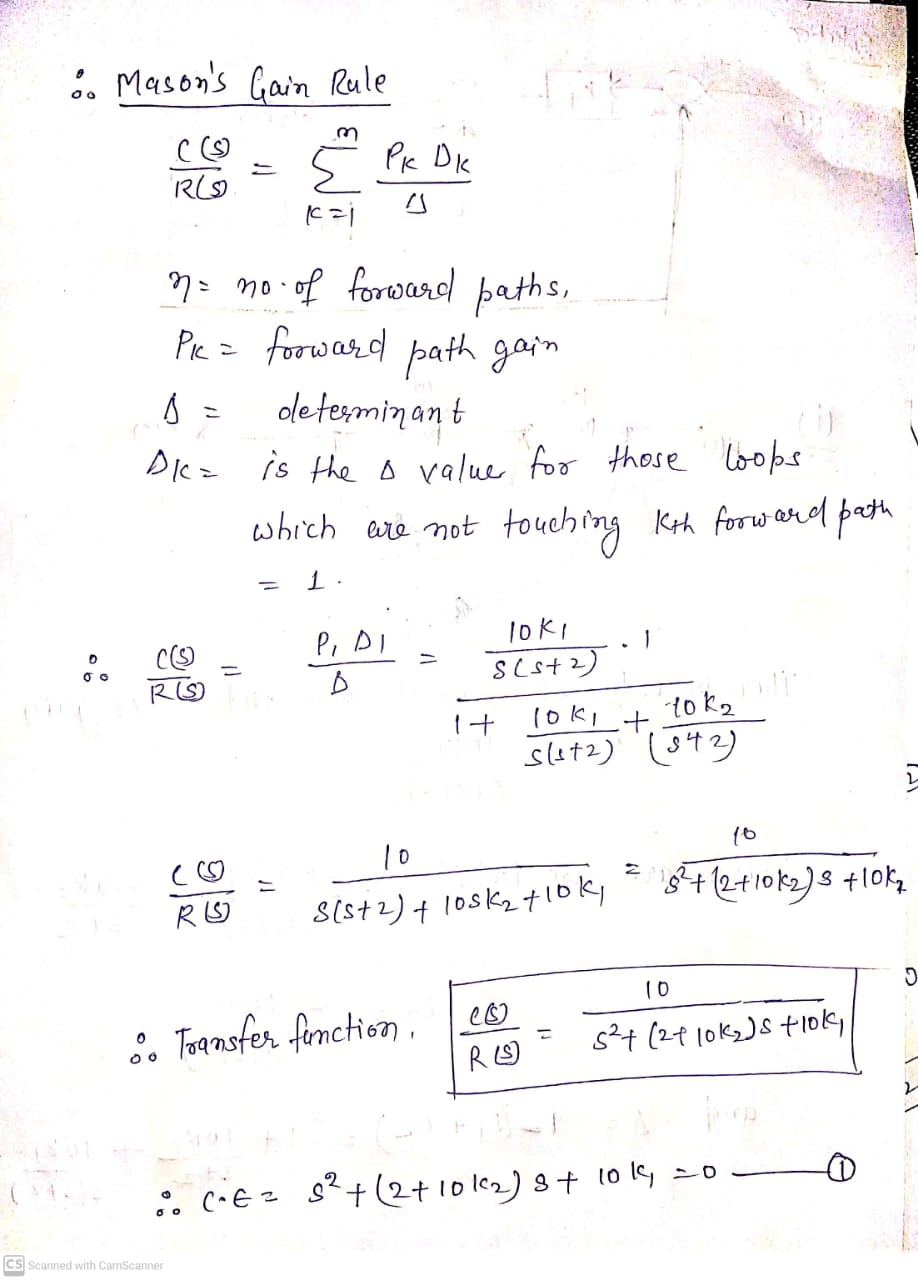Earn Coins

Coins can be redeemed for fabulous gifts.

Similar Homework Help Questions
• In feedback and control systems. Convert to signal flow graph and then apply mason's rule C(s)...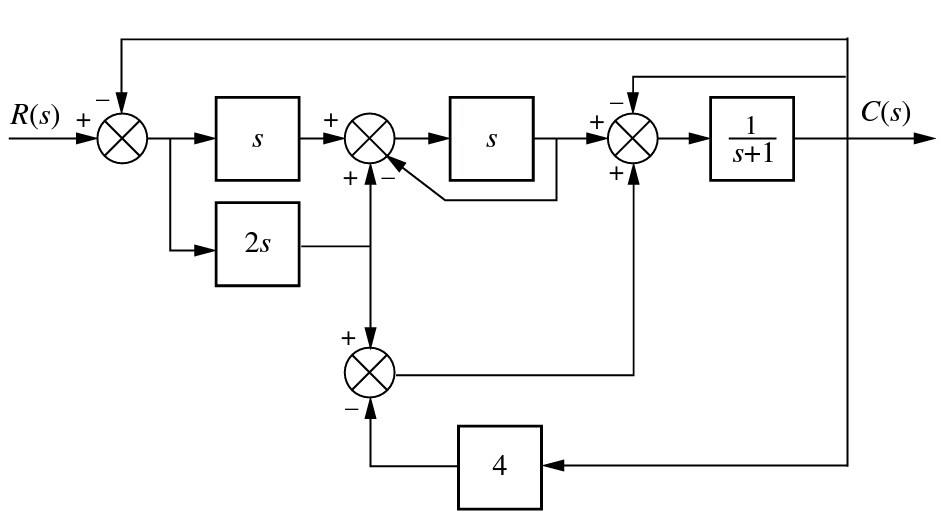In feedback and control systems. Convert to signal flow graph and then apply mason's rule C(s) R(s)+ s+l 4

• 4)Convert the following block diagram into signal flow graph (15 marks) R(s) X (s) U(s) H.(s)...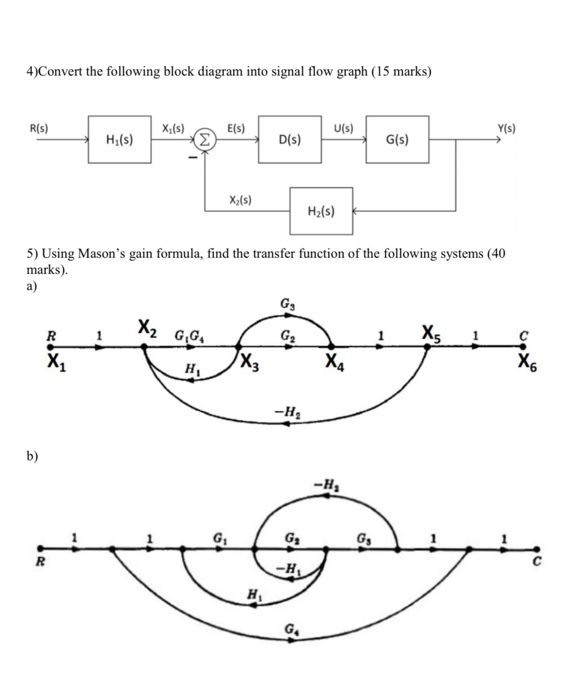4)Convert the following block diagram into signal flow graph (15 marks) R(s) X (s) U(s) H.(s) D(s) G(s) Xx(s) Hz(s) 5) Using Mason's gain formula, find the transfer function of the following systems (40 marks).

• Question 2 Figure 2 represents block diagram and signal flow chart which are commonly used in...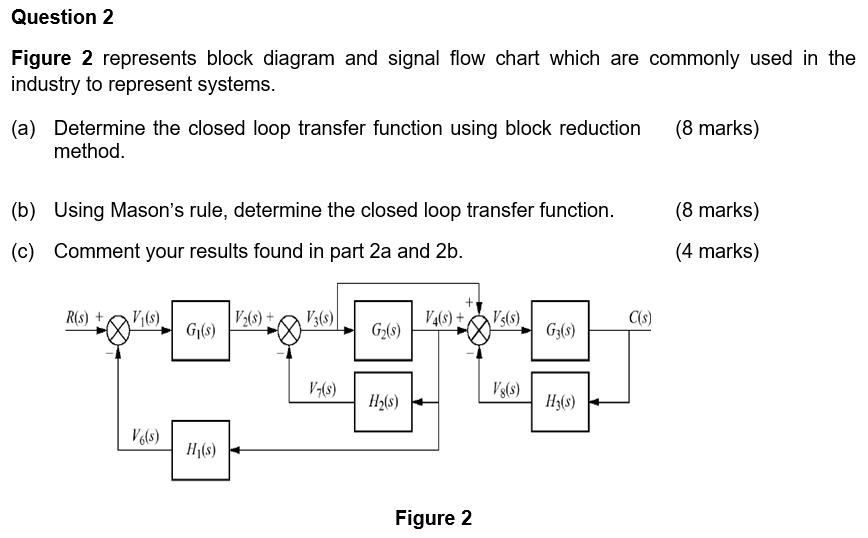Question 2 Figure 2 represents block diagram and signal flow chart which are commonly used in the industry to represent systems. (a) Determine the closed loop transfer function using block reduction method. (8 marks) (8 marks) (b) Using Mason's rule, determine the closed loop transfer function. (c) Comment your results found in part 2a and 2b. (4 marks) R(s) + Vi(s) V2() V3 (8) V4(s) + V:(s) C(s) G(S) Gz() G3(s) V-(8) H2(S) Hz(5) V (5) H (8) Figure 2

• Question: CODE: >> %% PID controller design Kp = 65.2861; Ki = 146.8418; Kd = 4.0444;...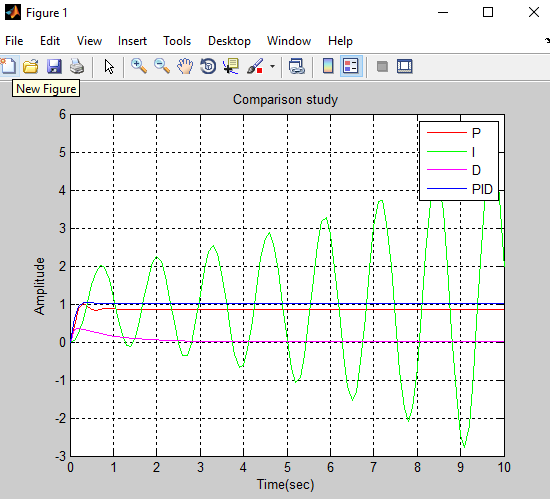Question: CODE: >> %% PID controller design Kp = 65.2861; Ki = 146.8418; Kd = 4.0444; Gc = pid(Kp,Ki,Kd); % close-loop TF T = feedback(G*Gc,1); %% checking the design obejective a_pid = stepinfo(T); % Settling Time tp_pid = a_pid.SettlingTime % Overshhot OS_pid = a_pid.Overshoot %% steady-state error [yout_pid,tout_pid] = lsim(T,stepInput,t); % steady-state error ess_pid = stepInput(end) - yout_pid(end); >> %% Effect of P in G Kp = 65.2861; Ki = 0; Kd = 0; Gc = pid(Kp,Ki,Kd); % close-loop TF...

• I have no more posting for this month, please solve these for me thanks 1. Given...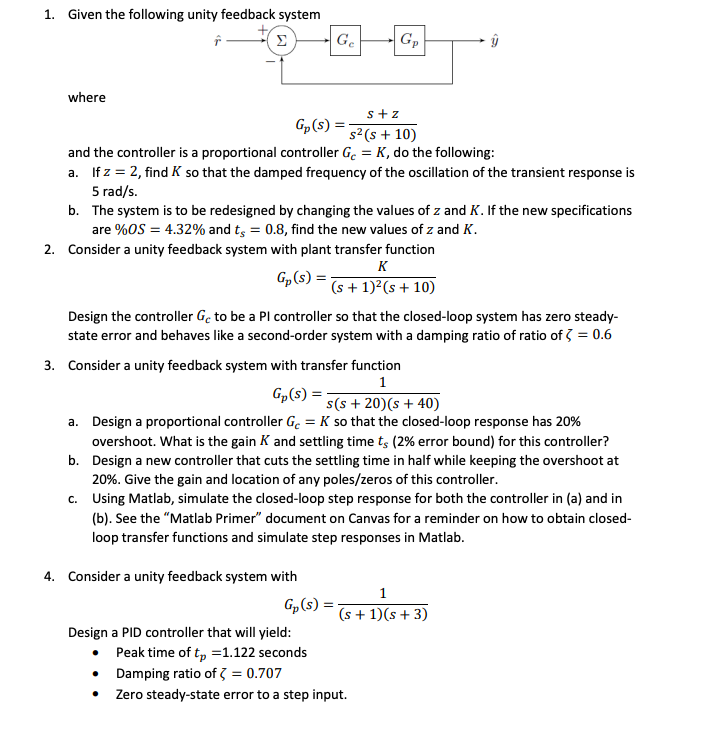I have no more posting for this month, please solve these for me thanks 1. Given the following unity feedback system where s+z s2 (s + 10) and the controller is a proportional controller Ge = K, do the following: a. If z = 2, find K so that the damped frequency of the oscillation of the transient response is 5 rad/s. b. The system is to be redesigned by changing the values of z and K. If the new...

• Question three The figure below shows a unit step response of a second order system. From...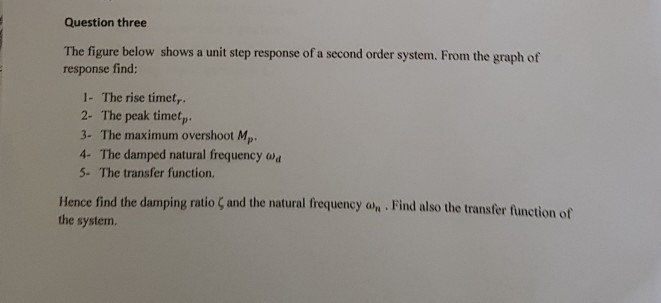Question three The figure below shows a unit step response of a second order system. From the graph of response find: 1- The rise timet, 2- The peak timet, 3- The maximum overshoot Mp 4- The damped natural frequency w 5. The transfer function. Hence find the damping ratio ζ and the natural frequency ah-Find also the transfer function of the system. r 4 02 15 25 35 45 Question Four For the control system shown in the figure below,...

• Hi can you help me solve the following signal and system question on CTFS harmonic functions. Please show me how to get Cx[K] by integration by parts step by step. The graph for this question is not n...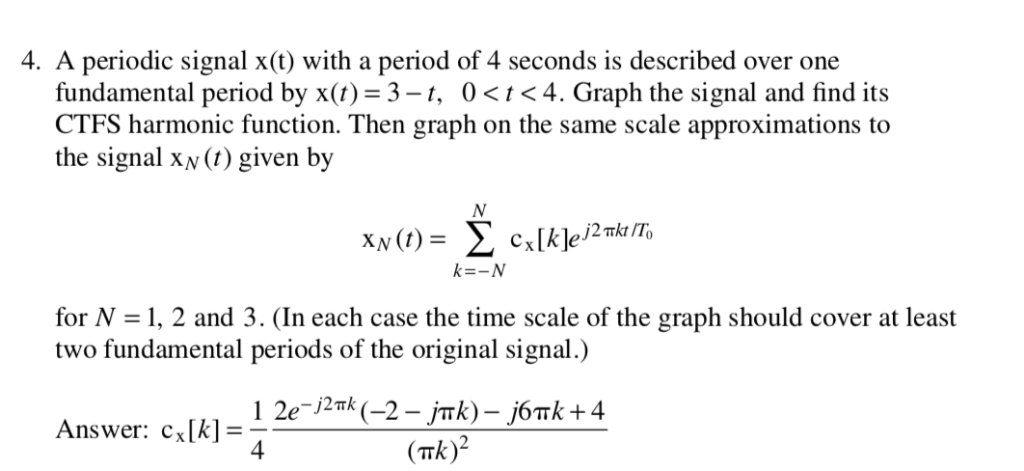Hi can you help me solve the following signal and system question on CTFS harmonic functions. Please show me how to get Cx[K] by integration by parts step by step. The graph for this question is not needed. Thank you. 4. A periodic signal x(t) with a period of 4 seconds is described over one fundamental period by x(t) 3-t, 0<t<4. Graph the signal and find its CTFS harmonic function. Then graph on the same scale approximations to the signal...

• Control Assignment 2017/18 In order to complete this assignment you will have to consider a system...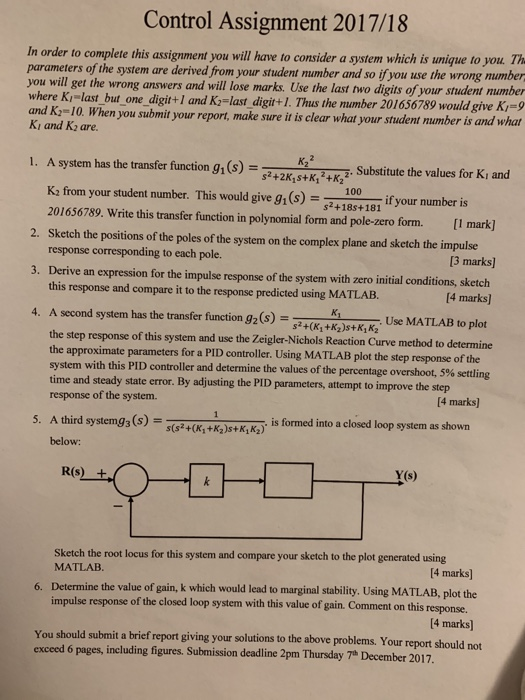Control Assignment 2017/18 In order to complete this assignment you will have to consider a system which is unique to you. Th parameters of the system are derived from your student mumber and so if you use the wrong mumber you will get the wrong answers and will lose marks. Use the last two digits of your student mumber where Ki-last but_one_digit+I and K-last digit+1. Thus the mumber 201656789 would give K9 and K 10. When you submit your report,...